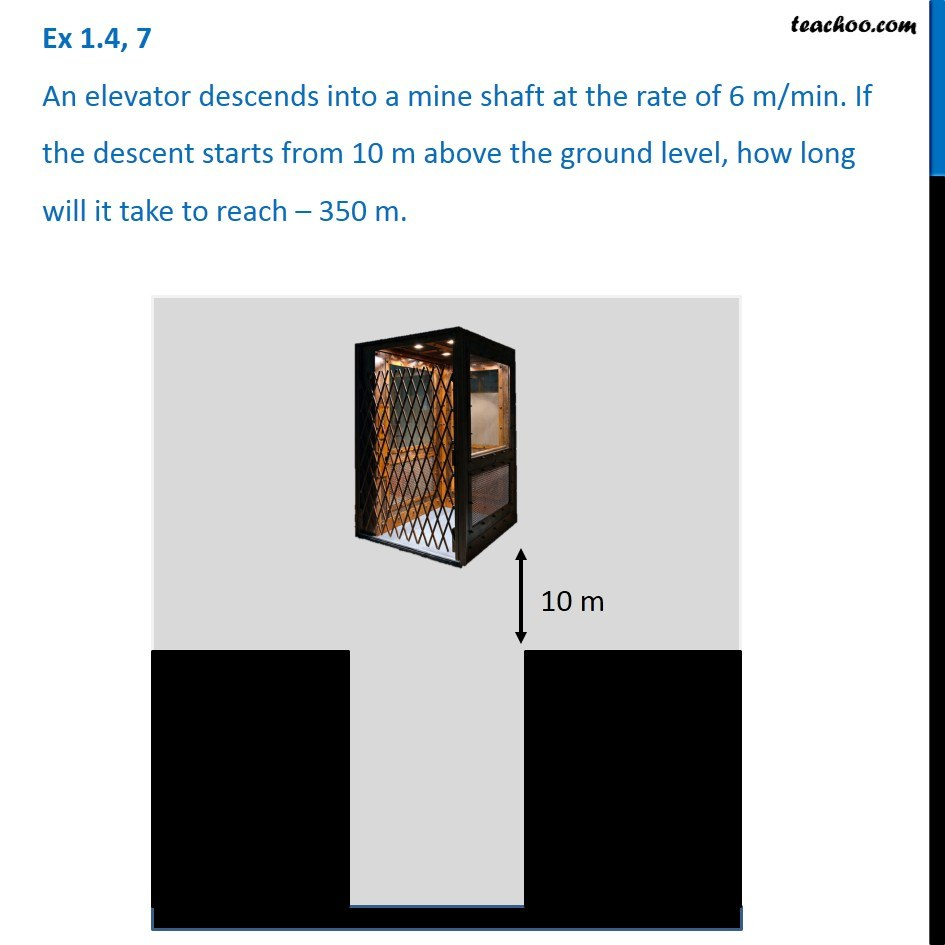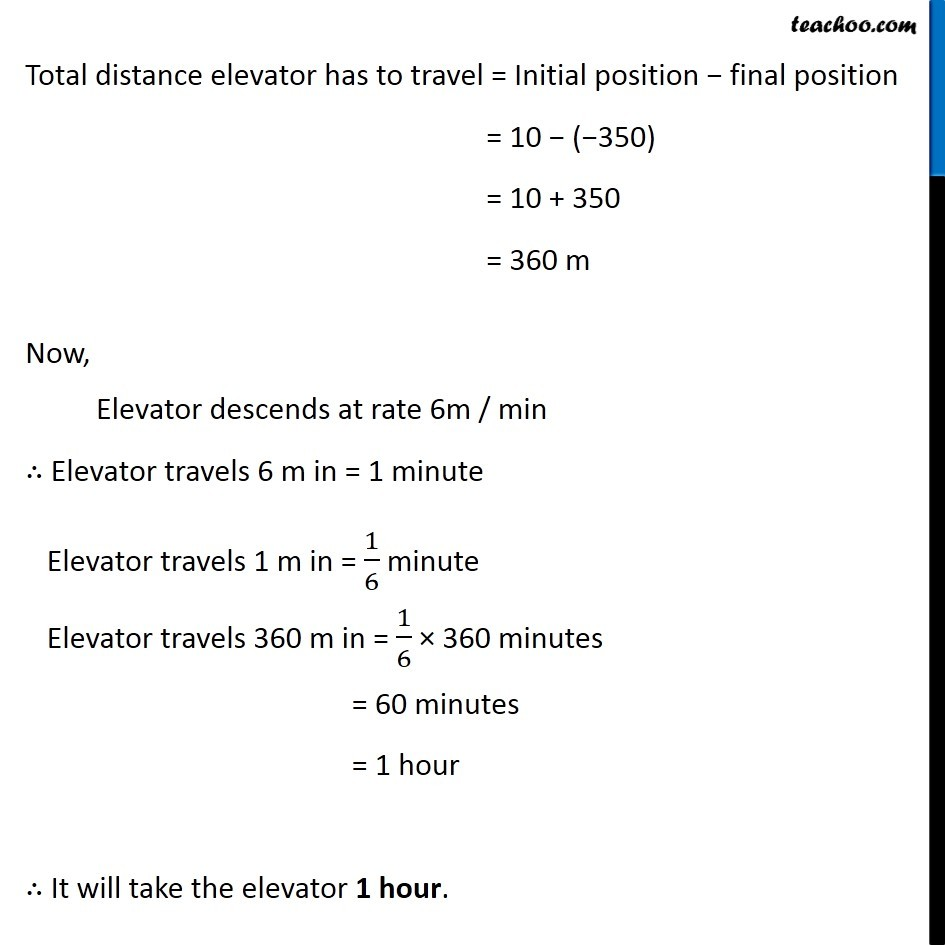1. Chapter 1 Class 7 Integers
2. Concept wise
3. Statement Questions - Division

Transcript

Ex 1.4, 7 An elevator descends into a mine shaft at the rate of 6 m/min. If the descent starts from 10 m above the ground level, how long will it take to reach 350 m. Total distance elevator has to travel = Initial position final position = 10 ( 350) = 10 + 350 = 360 m Now, Elevator descends at rate 6m / min Elevator travels 6 m in = 1 minute Elevator travels 1 m in = 1/6 minute Elevator travels 360 m in = 1/6 360 minutes = 60 minutes = 1 hour It will take the elevator 1 hour.